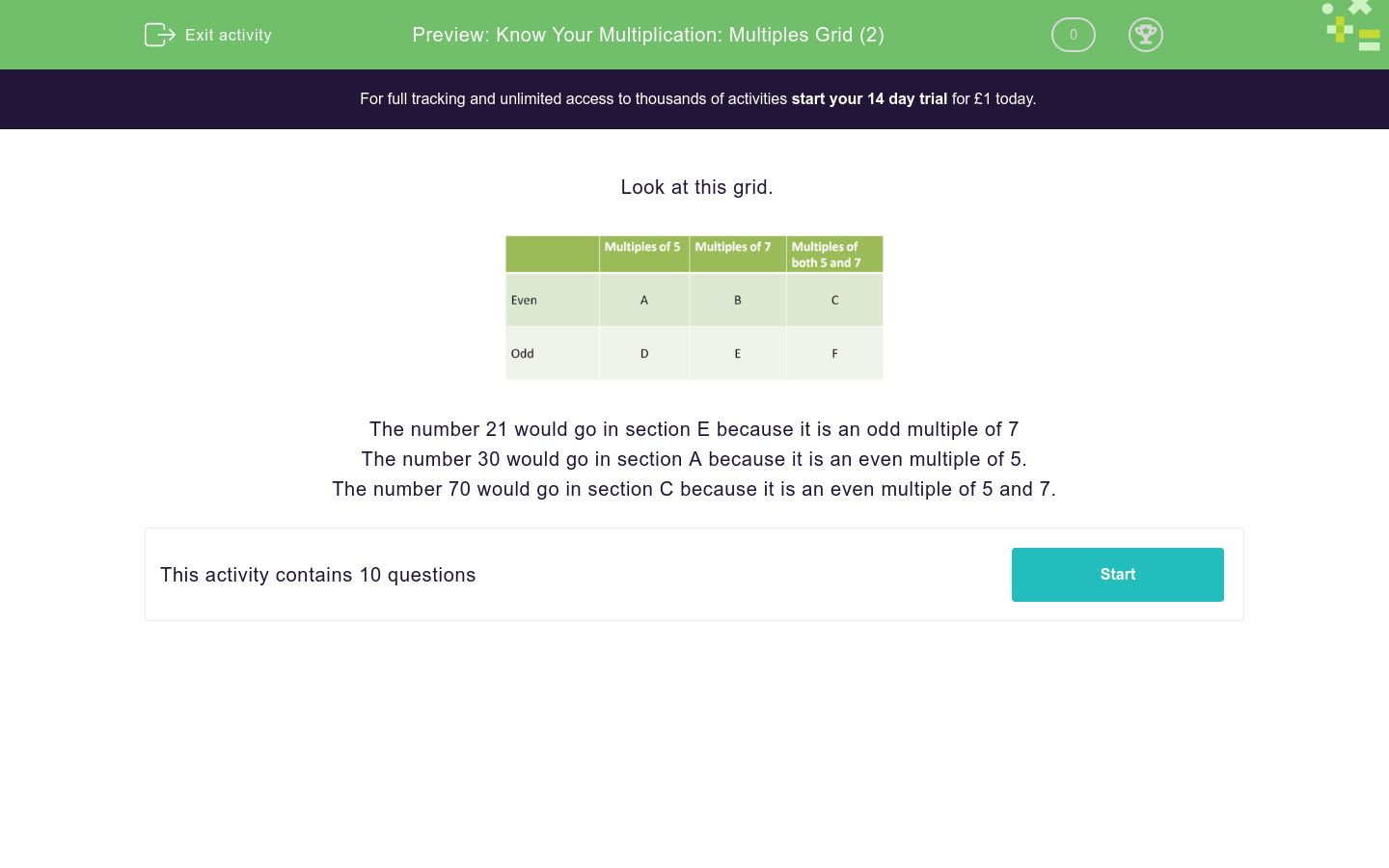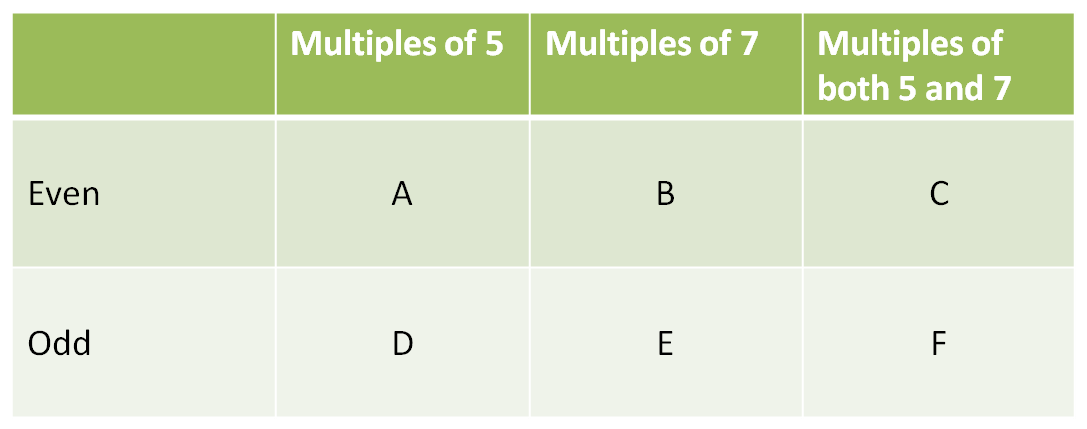# Know Your Multiplication: Multiples Grid (2)

In this worksheet, students decide where to place numbers in a grid of odd and even multiples.Key stage:  KS 2

Curriculum topic:   Number: Multiplication and Division

Curriculum subtopic:   Solve Multiplication Problems

Difficulty level:### QUESTION 1 of 10

Look at this grid.The number 21 would go in section E because it is an odd multiple of 7

The number 30 would go in section A because it is an even multiple of 5.

The number 70 would go in section C because it is an even multiple of 5 and 7.

In which section should the following number be placed on this grid.

21A

B

C

D

E

F

In which section should the following number be placed on this grid.

20A

B

C

D

E

F

In which section should the following number be placed on this grid.

14A

B

C

D

E

F

In which section should the following number be placed on this grid.

35A

B

C

D

E

F

In which section should the following number be placed on this grid.

25A

B

C

D

E

F

In which section should the following number be placed on this grid.

140A

B

C

D

E

F

In which section should the following number be placed on this grid.

90A

B

C

D

E

F

In which section should the following number be placed on this grid.

28A

B

C

D

E

F

In which section should the following number be placed on this grid.

56A

B

C

D

E

F

In which section should the following number be placed on this grid.

105A

B

C

D

E

F

• Question 1

In which section should the following number be placed on this grid.

21E
• Question 2

In which section should the following number be placed on this grid.

20A
• Question 3

In which section should the following number be placed on this grid.

14B
• Question 4

In which section should the following number be placed on this grid.

35F
• Question 5

In which section should the following number be placed on this grid.

25D
• Question 6

In which section should the following number be placed on this grid.

140C
• Question 7

In which section should the following number be placed on this grid.

90A
• Question 8

In which section should the following number be placed on this grid.

28B
• Question 9

In which section should the following number be placed on this grid.

56B
• Question 10

In which section should the following number be placed on this grid.

105F
---- OR ----

Sign up for a £1 trial so you can track and measure your child's progress on this activity.

### What is EdPlace?

We're your National Curriculum aligned online education content provider helping each child succeed in English, maths and science from year 1 to GCSE. With an EdPlace account you’ll be able to track and measure progress, helping each child achieve their best. We build confidence and attainment by personalising each child’s learning at a level that suits them.

Get started